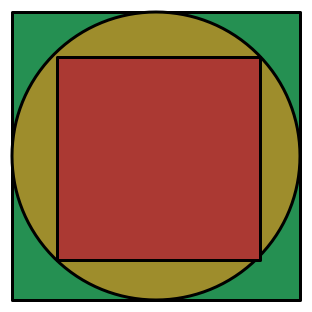Square In Circle in SquareIn a large square, the incircle is drawn (with diameter equal to the side length of the large square).

In the circle, a smaller square is inscribed.

What is the ratio of the area of the smaller square to the area of the larger square?

×